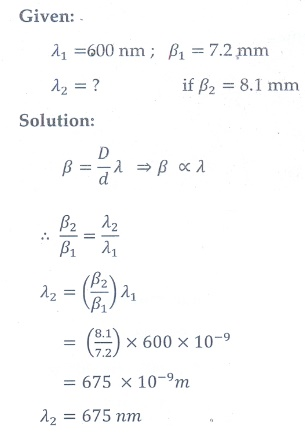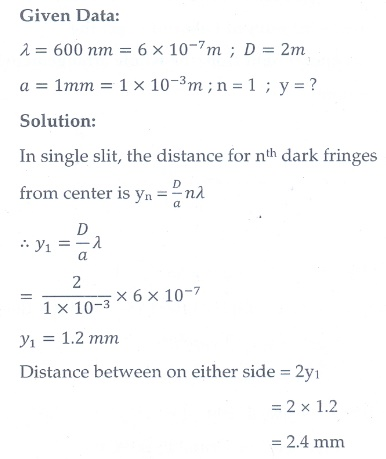Home | | Physics 12th Std | Book Back Numerical Problems

# Book Back Numerical Problems

Physics : Wave Optics: Book Back, Exercise, Example Numerical Question with Answers, Solution

Numerical Problems

1. The ratio of maximum and minimum intensities in an interference pattern is 36:1. What is the ratio of the amplitudes of the two interfering waves?

[Ans: 7:5]2. In Young’s double slit experiment, 62 fringes are seen in visible region for sodium light of wavelength 5893 Å. If violet light of wavelength 4359 Å is used in place of sodium light, then what is the number of fringes seen? [Ans: 84]3. Light of wavelength 600 nm that falls on a pair of slits producing interference pattern on a screen in which the bright fringes are separated by 7.2 mm. What must be the wavelength of another light which produces bright fringes separated by 8.1 mm with the same apparatus?

[Ans: 675 nm]4. A beam of light of wavelength 600 nm from a distant source falls on a single slit 1 mm wide and the resulting diffraction pattern is observed on a screen 2 m away. What is the distance between the first dark fringe on either side of the central bright fringe?

[Ans: 2.4 mm]5. Light of wavelength of 5000 Å produces diffraction pattern of the single slit of width 2.5 µm. What is the maximum order of diffraction possible?

[Ans: 5]6. I0 is the intensity of light existing between two cross Polaroids kept with their axes perpendicular to each other. A third polaroid is introduced between them. What must be the angle between the axes of first and the newly introduced polaroid to ge the maximum light from the whol arrangement?

[Ans: 45°]7. An unpolarised light of intensity 32 Wm-2 passes through three Polaroids such that the axes of the first and the last Polaroids are at 90°. What is the angle between the axes of the first and middle Polaroids so that the emerging light has an intensity of only 3 Wm-²?

[Ans: 30°]8. The reflected light is found to be plane polarised when an unpolarized light falls on a denser medium at 60° with the normal. Find the angle of refraction and critical angle of incidence for total internal reflection in the denser to rarer medium reflection.

[Ans: 30°, 35.15°]9. The near point and the far point for a person are 50 cm and 500 cm, respectively. Calculate the power of the lens the person should wear to read a book held in hand at 25 cm. What maximum distance is clearly visible for the person with this lens on the eye?

[Ans: 2D, 45.45 cm]10. A compound microscope has a magnifying power of 100 when the image is formed at infinity. The objective has a focal length of 0.5 cm and the tube length is 6.5 cm. What is the focal length of the eyepiece.

[Ans: 2 cm]Tags : with Answer and Solution | Wave Optics | Physics , 12th Physics : UNIT 7 : Wave Optics
Study Material, Lecturing Notes, Assignment, Reference, Wiki description explanation, brief detail
12th Physics : UNIT 7 : Wave Optics : Book Back Numerical Problems | with Answer and Solution | Wave Optics | Physics